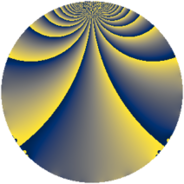# Properties

 Label 1805.2.iLevel $1805$ Weight $2$ Character orbit 1805.i Rep. character $\chi_{1805}(429,\cdot)$ Character field $\Q(\zeta_{6})$ Dimension $308$ Sturm bound $380$

# Related objects

## Defining parameters

 Level: $$N$$ $$=$$ $$1805 = 5 \cdot 19^{2}$$ Weight: $$k$$ $$=$$ $$2$$ Character orbit: $$[\chi]$$ $$=$$ 1805.i (of order $$6$$ and degree $$2$$) Character conductor: $$\operatorname{cond}(\chi)$$ $$=$$ $$95$$ Character field: $$\Q(\zeta_{6})$$ Sturm bound: $$380$$

## Dimensions

The following table gives the dimensions of various subspaces of $$M_{2}(1805, [\chi])$$.

Total New Old
Modular forms 420 372 48
Cusp forms 340 308 32
Eisenstein series 80 64 16

## Trace form

 $$308 q + 138 q^{4} - q^{5} + 12 q^{6} + 124 q^{9} + O(q^{10})$$ $$308 q + 138 q^{4} - q^{5} + 12 q^{6} + 124 q^{9} + 2 q^{10} - 4 q^{11} - 6 q^{14} + 4 q^{15} - 102 q^{16} - 12 q^{20} + 20 q^{21} + 6 q^{24} - q^{25} + 76 q^{26} - 6 q^{29} - 12 q^{30} - 32 q^{31} - 2 q^{34} - 13 q^{35} - 106 q^{36} - 76 q^{39} - 10 q^{40} + 16 q^{41} - 16 q^{44} - 58 q^{45} - 56 q^{46} - 48 q^{49} + 40 q^{50} + 40 q^{51} + 12 q^{54} + 29 q^{55} - 92 q^{56} - 38 q^{59} - 4 q^{60} - 14 q^{61} - 128 q^{64} + 24 q^{65} - 110 q^{66} + 36 q^{69} - 62 q^{70} + 14 q^{74} + 56 q^{75} - 26 q^{79} - 16 q^{80} + 14 q^{81} + 96 q^{84} - 15 q^{85} - 8 q^{86} - 14 q^{89} + 32 q^{90} - 8 q^{91} + 108 q^{94} - 76 q^{96} + 30 q^{99} + O(q^{100})$$

## Decomposition of $$S_{2}^{\mathrm{new}}(1805, [\chi])$$ into newform subspaces

The newforms in this space have not yet been added to the LMFDB.

## Decomposition of $$S_{2}^{\mathrm{old}}(1805, [\chi])$$ into lower level spaces

$$S_{2}^{\mathrm{old}}(1805, [\chi]) \cong$$ $$S_{2}^{\mathrm{new}}(95, [\chi])$$$$^{\oplus 2}$$